## "Pressure Head Fractal Dimension for Characterizing Shajara Reservoirs of the Shajara Formation of the Permo-Carboniferous Unayzah Group, Saudi Arabia"

Khalid Elyas Mohamed Elamin AlKhidir*

Department of Petroleum Engineering and Natural Gas, King Saud University, Saudi Arabia

*Corresponding author: Khalid Elyas Mohamed Elamin AlKhidir, Department of Petroleum Engineering and Natural Gas, College of Engineering, King Saud University, Riyadh, Saudi Arabia. Tel: +96611 4679118; Email: kalkhidir@ksu.edu.sa

Received Date: 27 June, 2017; Accepted Date: 13 July, 2017; Published Date: 20 July, 2017

1.                   Abstract

Pressure head was gained from distribution of pores to characterize the sandstones of the Shajara reservoirs of the Shajara Formation of the Permo-Carboniferous Unayzah Group. The attained values of pressure head were employed to calculate the pressure head fractal dimension. Based on field observations in addition to the acquired values of pressure head fractal dimension, the sandstones of Shajara reservoirs were divided here into three units. The obtained units from base to top are: Lower Shajara Pressure Head Fractal Dimension Unit, Middle Shajara Pressure Head Fractal Dimension Unit and Upper Shajara Pressure Head Fractal Dimension Unit.

The three Shajara reservoirs were also confirmed by the ratio of bubble pressure to capillary pressure at other points versus effective saturation. It was reported that the permeability accelerates with increasing bubble pressure fractal dimension due to the naturally occurring interconnected channels. The pressure head fractal dimension and bubble pressure fractal dimension was successfully characterize the sandstones of the Shajara reservoirs with certain degree of accuracy.

1.                   Introduction

Capillary pressure is generally expressed as an aspect of the wetting phase saturation, according   to the capillary pressure model . The capillary pressure function was modified by Brooks and Corey (1964)  by applying a pore size distribution index (λ) as an exponent on the ratio of bubble pressure to capillary pressure. According to their results, a linear relationship exists between pressure and effective saturation on a log-log plot. This mathematical relationship has been named the Brooks-Corey model (B-C model). A model that predicts the hydraulic conductivity for un- saturated soil-water retention curve and conductivity saturation was derived by Mualem (1976) . Later, based on Mualem's formula, Van Genuchten (1980)  described a relatively simple expression for the hydraulic conductivity of unsaturated soils. The Van Genuchten model (V-G model) contained three independent parameters, which can be obtained by fitting experimental data. A function to estimate the relationship between water saturation and capillary pressure in porous media was proposed by Oostrom and Lenhard (1998) . This function is test data of sandstone rocks and carbonate rocks with high permeability were described by a new capillary pressure expression by Jing and Van Wunnik (1998) .

A fractal approach can be used to model the pc measured with mercury intrusion in Geysers grey wackerock; however, the B-C model could not be used, according to a study by Li (2004) . Sub-sequently, a theoretical analysis using fractal geometry was conducted by Li and Horne (2006)  to deduce the B-C model, which has always been considered as an empirical model. Subsequently, fractal modeling of porous media was used to develop a more generalized capillary pressure model (Li, 2010a) . With the new model, he also evaluated the heterogeneity of rocks (Li, 2010b) . Al-Khidir, et al. 2011  studied Bimodal Pore Size behavior of the Shajara Formation reservoirs of the permo-carboniferous Unayzah group. Al-Khidir, et al. (2012)  subdivided the Shajara reservoirs into three units based on thermodynamic fractal dimension approach and 3-D fractal geometry model of mercury intrusion technique. The work published by Al-Khidir, et al. 2012 was cited as Geoscience; New Finding reported from King Saud University Describe advances in Geoscience. Science Letter (Oct 25, 2013): 359. Al-khidir, et al. 2013 [13,14] subdivide the Shajara reservoirs into three units: Lower Shajara Differential Capacity Fractal Dimension Unit, Middle Shajara Differential Capacity Fractal Dimension Unit, Upper Shajara Differential Capacity Fractal Dimension Unit. The Three reservoirs units were confirmed by water saturation fractal dimension. Al-Khidir 2015  subdivided the Shajara reservoirs into three induced polarization geometric time fractal dimension units and confirmed them by arithmetic relaxation time fractal dimension of induced polarization.

2.       Materials and Methods

The purpose of this paper is to obtain pressure head fractal dimension (Dαh) and to confirm it by bubble pressure fractal dimension (DP/pc). The pressure head fractal dimension is determined from the slope of the plot of effective wetting phase saturation (Se) versus pressure head (α*h). The exponent on the pressure head relates to the fitting parameters m*n which match with the pore size distribution index (λ). The bubble pressure fractal dimension was obtained from the slope of the plot of wetting phase effective saturation (Se) versus the plot of the ratio of bubbling pressure (Pb) to capillary pressure (pc). The slope of the functional relationships among capillary pressure and effective saturation correspond to the pore size distribution index (λ). Theoretically, the pore size distribution index being small for media having a wide range of pore sizes and large for media with a relatively uniform pore size.

Field Data: Sandstone samples were collected from the type section of the Shajara Formation of the Permo-Carboniferous Unayzah Group, Wadi Shajara, Qusayba area, al Qassim district, Saudi Arabia, N 2652 17.4, E 4336 18. (Figure1). Porosity was measured on collected samples using mercury intrusion Porosimetry and permeability was derived from capillary pressure data.

Symbols: Φ porosity in percent, K permeability in millidarcy, λ pore size distribution index, m, n, are fitting parameter, Pb bubble pressure in psi, pc capillary pressure in psi, α inverse of pressure head in cm, h is the pressure head in cm, Dp/pc.  Bubble pressure fractal dimension, Dαh pressure head fractal dimension, Se effective wetting phase saturation.

3.       Results

The obtained results of the log log plot of the ratio of bubble pressure (Pb) to pressure (p) and the product of inverse pressure head (α) and pressure head (h) versus effective wetting phase saturation (Se) are shown in (Figures 2: Figure 11). A straight line was attained whose slope is equal to the pore size distribution index (λ) of Brooks and Corey (1964)  and the fitting parameters m times n of Van Genuchten (1980)  respectively. Based on the acquired results it was found that the pore size distribution index is equal to the fitting parameters m*n. The maximum value of the pore size distribution index was found to be 0.4761 assigned to sample SJ5 from the Lower Shajara Reservoir (Table 1). Whereas the minimum value of the pore size distribution index was reported from sample SJ13 from the Upper Shajara reservoir (Table 1). The pore size distribution index and the fitting parameters m*n were observed to decrease with increasing permeability (Table 1) owing to the possibility of having interconnected channels.

The bubble pressure fractal dimension and pressure head fractal dimension which were derived from the pore size index were noticed to increase with increasing permeability and show similarity in their values (Table 1). Based on field observation the Shajara Reservoirs of the Shajara Formation of the Permo-Carboniferous Unayzah Group were divided into three units (Figure 1).

These units from base to top are: Lower Shajara Reservoir, Middle Shajara reservoir, and Upper Shajara Reservoir. The Lower Shajara reservoir was represented by four sandstone samples out of six, namely SJ1, SJ2, SJ3 and SJ4.Sample SJ1 is described as medium-grained, porous, permeable, and moderately well sorted red sandstone (Figure 1). Its pore size distribution index and fitting parameter m*n show similar values (Figure 2 and Table 1)

Its bubble pressure fractal dimension and pressure head fractal dimension which was derived from pore size distribution index and the fitting parameter m*n respectively also indicates similar values (Table 1). (Figure 3) delineates straight line plot of log (Pb/p) versus log effective saturation, and log (α*h) versus log effective saturation of sample SJ2 which is defined as medium - grained, porous, permeable, well sorted, yellow sandstone (Figure 1). It acquired a pore size distribution index of about 0.2252 whose value equal to m*n (Figure 3).

It is also characterized by similarity in bubble pressure fractal dimension and pressure head fractal dimension as displayed in (Table 1). As we proceed from sample SJ2 to SJ3 a pronounced reduction in permeability due to compaction was reported from 1955 md to 56 md which reflects an increase in pore size distribution index from0.2252 to 0.5621 and reduction in fractal dimension from 2.7748 to 2.4379 as stated in (Table 1). Again, an increase in grain size and permeability was recorded from sample SJ4 which is characterized by 0.3157 pore size distribution index and 2.6843 bubble pressure fractal dimension which agree with the pressure head fractal dimension (Table 1). In contrast, the Middle Shajara reservoir which is separated from the Lower Shajara reservoir by an unconformity surface (Figure 1) was designated by three samples out of four, namely SJ7, SJ8, and SJ9 as illustrated in (Figure 1). Their pore size distribution index and fitting parameters m*n were reported in (Figures 6, 7, 8, and Table 1).

Their bubble pressure fractal dimensions and pressure head fractal dimensions are higher than those of samples SJ3 and SJ4 from the Lower Shajara Reservoir due to an increase in their permeability (Table 1). On the other hand, the Upper Shajara reservoir is separated from the Middle Shajara reservoir by yellow green mudstone as demonstrated in (Figure 1). It is defined by three samples so called SJ11, SJ12, SJ13 as explained in (Figure 1). Furthermore, their pore size distribution index and the fitting parameters m*n were demonstrated in (Figure 9,10,11, and Table 1).

Moreover, their bubble pressure fractal dimension and pressure head fractal dimension are also higher than those of sample SJ3 and SJ4 from the Lower Shajara Reservoir due to an increase in their flow capacity (permeability) as explained in (Table 1). Overall a plot of pore size distribution index and the fitting parameter n versus fractal dimension (Figure 12) reveals three permeable zones of varying Petrophysical properties. The higher fractal dimension zone with fractal dimension higher than 2.75 (Figure 12) corresponds to the full Upper Shajara Reservoir, entire Middle Shajara Reservoir and Sample SJ1 and SJ2 from the lower Shajara Reservoir. The middle fractal dimension zone with a value of about 2.68 (Figure12) resembles sample SJ4 from the lower Shajara reservoir.

The lower fractal dimension value2.43 allocates to sample SJ3 from the Lower Shajara reservoir as shown in (Figure 12). The three Shajara fractal dimension zones were also confirmed by plotting pressure head fractal dimension versus bubble pressure fractal dimension as illustrated in (Figure 13).

4.       Conclusion

1.                   The obtained Shajara bubble pressure fractal dimension reservoir units were also confirmed by pressure head fractal dimension.

2.                   It was found that, the higher the bubble pressure fractal dimension and pressure head fractal dimension, the higher the permeability leading to better Shajara reservoir characteristics.

3.                    It was also reported that, the bubble pressure fractal dimension and pressure head fractal dimension increases with decreasing pore size distribution index and fitting parameters m*n owing to possibility of having interconnected channels.

4.                   Digenetic features such as compaction plays an important role in reducing bubble pressure fractal dimension and pressure head fractal dimension due to reduction in pore connectivity.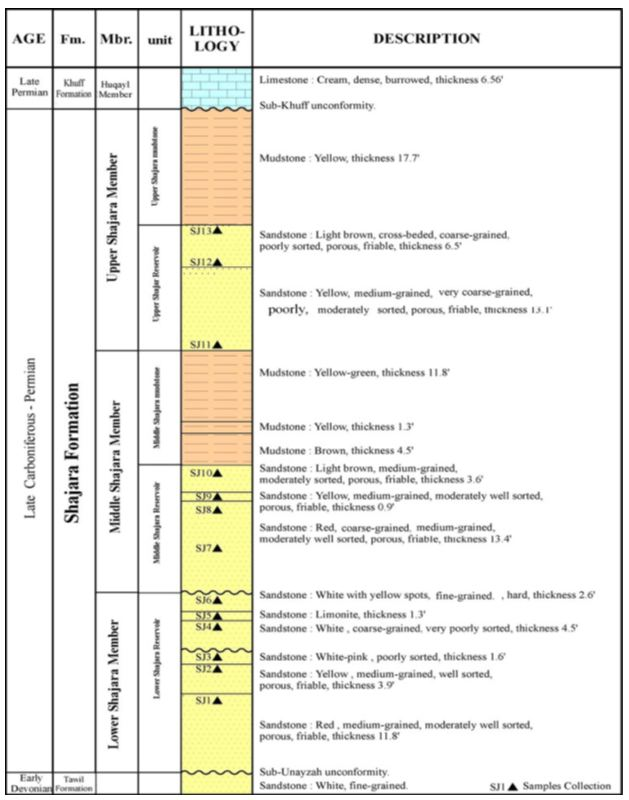Figure 1: Stratigraphic column of the type section of the Permo-Carboniferous Shajara Formation of the Unayzah Group, Wadi Shajara, Qusayba area, al Qassim district, Saudi Arabia, N 2652 17.4, E 4336 18.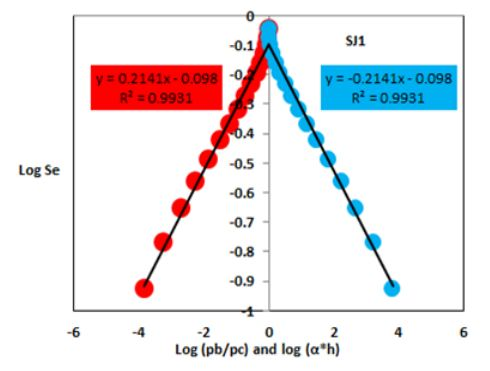Figure 2: Log (Pb/pc) versus log Se in red color and Log (α*h) versus log Se in blue Color.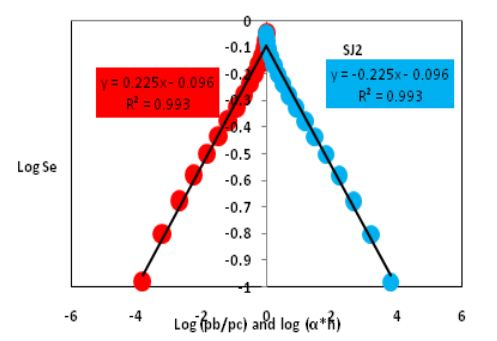Figure 3: Log (Pb/pc) versus log Se in red color and log (α*h) versus log Se in blue color.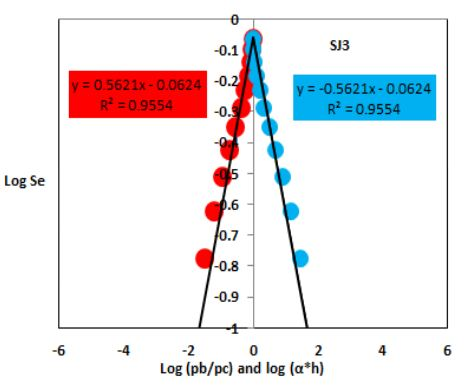Figure 4: Log (Pb/pc) versus log Se in red color and log (α*h) versus log Se in blue.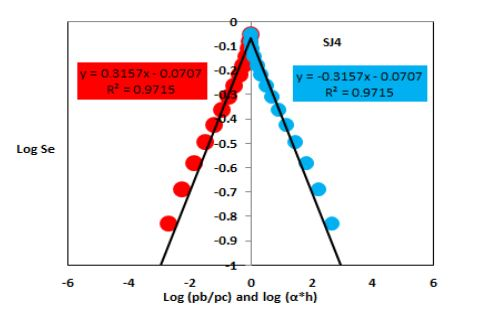Figure 5: Log (Pb/pc) versus log Se in red color and log (α*h) versus log Se in blue Color.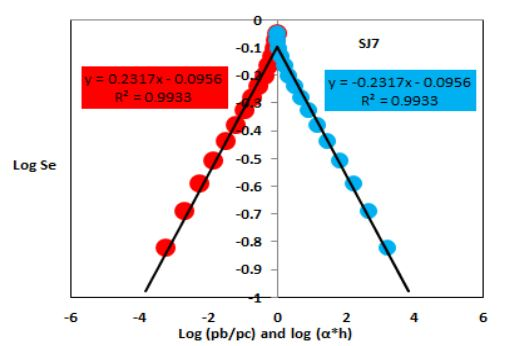Figure 6: Log (Pb/pc) versus log Se in red color and log (α*h) versus log Se in blue.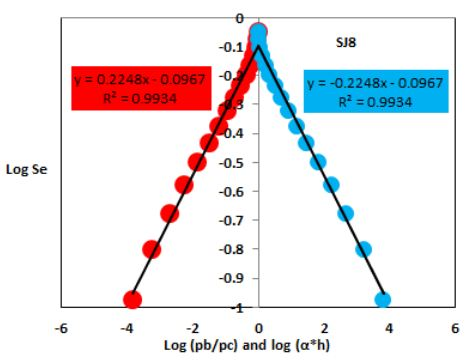Figure 7: Log (Pb/pc) versus log Se in red color and log (α*h) versus log Se in blue color.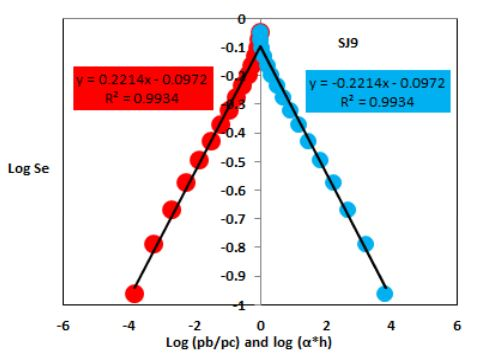Figure 8: Log (Pb/pc) versus log Se in red color and log (α*h) versus log Se in blue Color.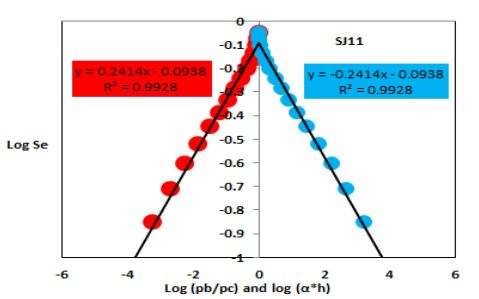Figure 9: Log (Pb/pc) versus log Se in red color and log (α*h) versus log Se in blue Color.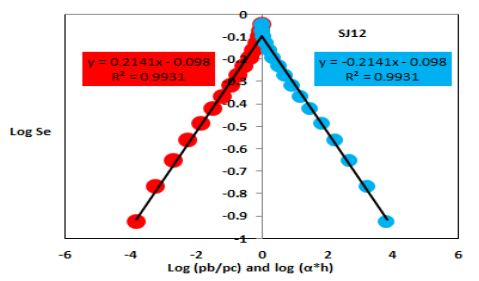Figure 10: Log (Pb/pc) versus log Se in red color and log (α*h) versus log Se in blue Color.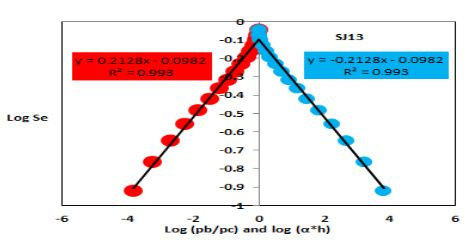Figure 11: Log (Pb/pc) versus log Se in red color and log (α*h) versus log Se in blue Color.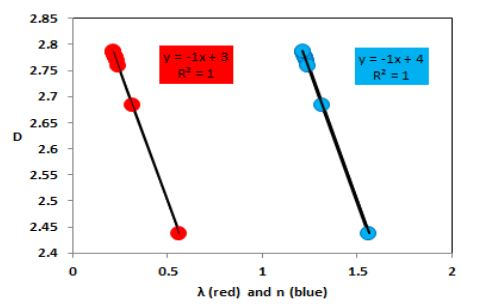Figure 12: Pore size distribution index (λ) in red color and fitting parameter (n) in blue color versus fractal Dimension (D).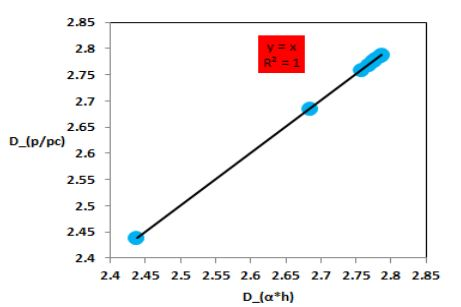Figure 13: Pressure head fractal dimension (Dαh) versus bubble pressure fractal dimension (DP/pc).

 Reservoirs Sample Φ (%) k (md) λ m*n m n Dp/pc Dαh Lower Shajara Reservoir SJ1 29 1680 0.2141 0.2141 0.176345 1.2141 2.7859 2.7859 SJ2 35 1955 0.2252 0.2252 0.183807 1.2252 2.7748 2.7748 SJ3 34 56 0.5621 0.5621 0.359836 1.5621 2.4379 2.4379 SJ4 30 176 0.3157 0.3157 0.239948 1.3157 2.6843 2.6843 Middle Shajara Reservoir SJ7 35 1472 0.2317 0.2317 0.188114 1.2317 2.7683 2.7683 SJ8 32 1344 0.2248 0.2248 0.18354 1.2248 2.7752 2.7752 SJ9 31 1394 0.2214 0.2214 0.181267 1.2214 2.7786 2.7786 Upper Shajara Reservoir SJ11 36 1197 0.2414 0.2414 0.194458 1.2414 2.7586 2.7586 SJ12 28 1440 0.2141 0.2141 0.176345 1.2141 2.7859 2.7859 SJ13 25 973 0.2128 0.2128 0.175462 1.2128 2.7872 2.7872

Table 1: Petrophysical properties characterizing Shajara reservoirs of the Shajara Formation of the Permo-Carboniferous Unayzah Group.

Citation: Al-Khidir KEME (2017) Pressure Head Fractal Dimension for Characterizing Shajara Reservoirs of the Shajara Formation of the Permo-Carboniferous Unayzagh Group, Saudi Arabia. Arch Pet Environ Biotechnol: APEB -113. DOI: 10.29011/2574-7614. 100113